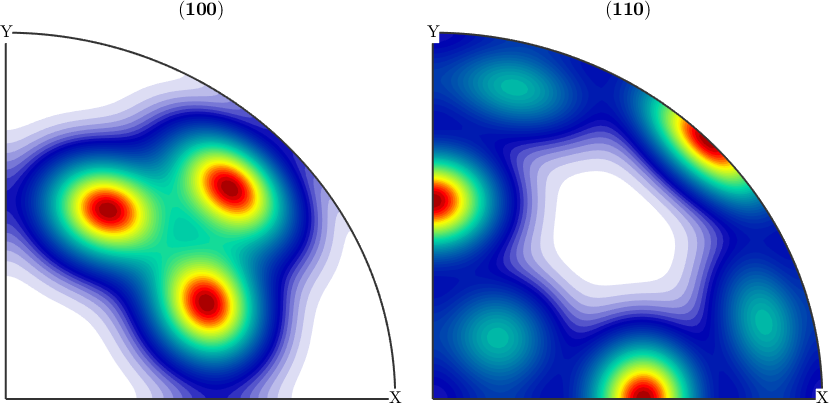ODF Modeling edit page

ODFs are functions on the rotation group $$SO(3)$$. Therefore we construct them by the class SO3Fun.

MTEX provides a very simple way to define model ODFs. Generally, there are five different ODF types in MTEX:

The central idea is that MTEX allows you to calculate mixture models, by adding and subtracting arbitrary ODFs. Model ODFs may be used as references for ODFs estimated from pole figure data or EBSD data and are instrumental for simulating texture evolutions.

## The Uniform ODF

The most simplest case of a model ODF is the uniform ODF

$f(g) = 1,\quad g \in SO(3),$

which is everywhere identical to one. In order to define a uniform ODF one needs only to specify its crystal and specimen symmetry and to use the command uniformODF.

## Combining model ODFs

All the above can be arbitrarily rotated and combined. For instance, the classical Santafe example can be defined by commands